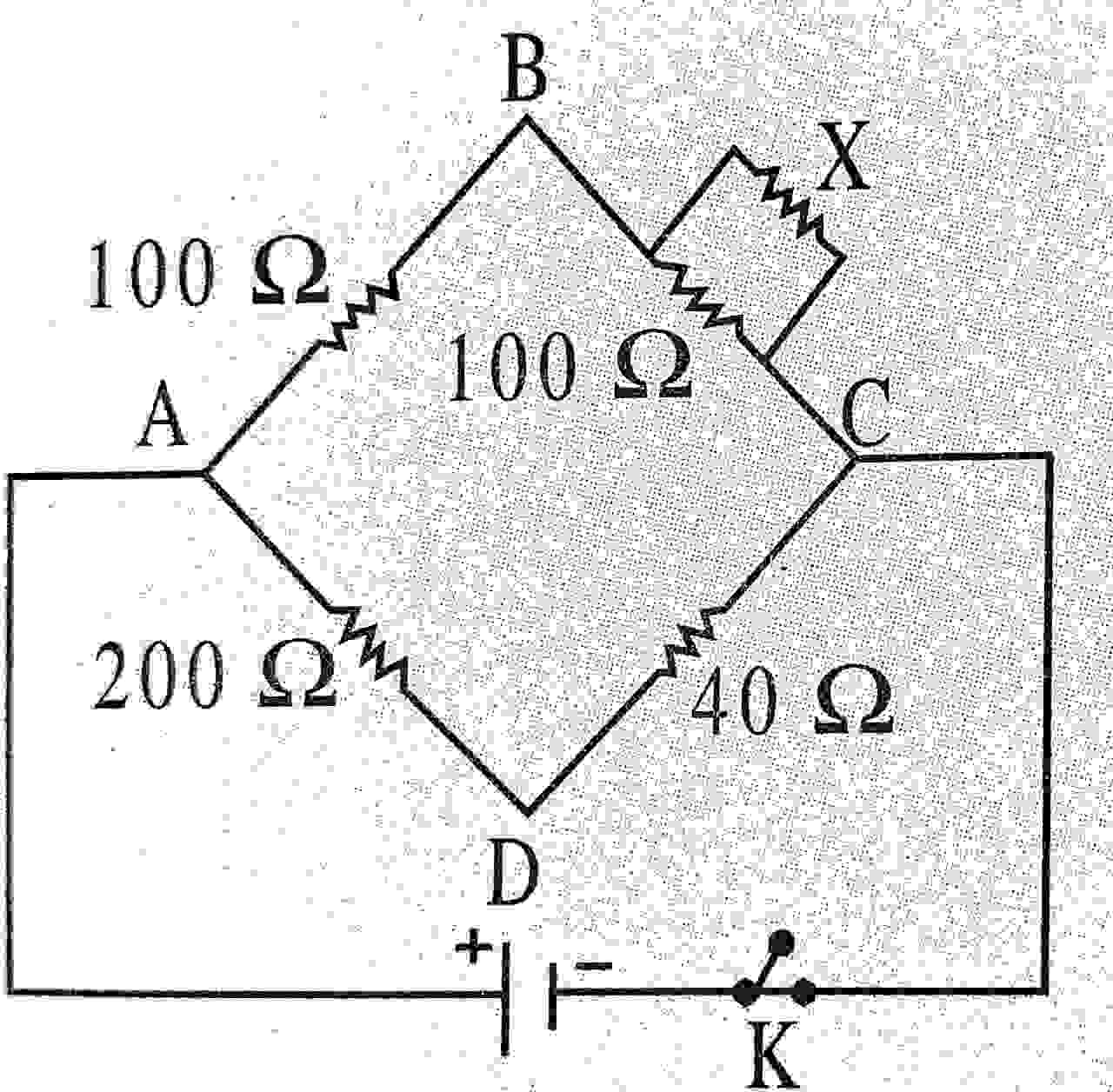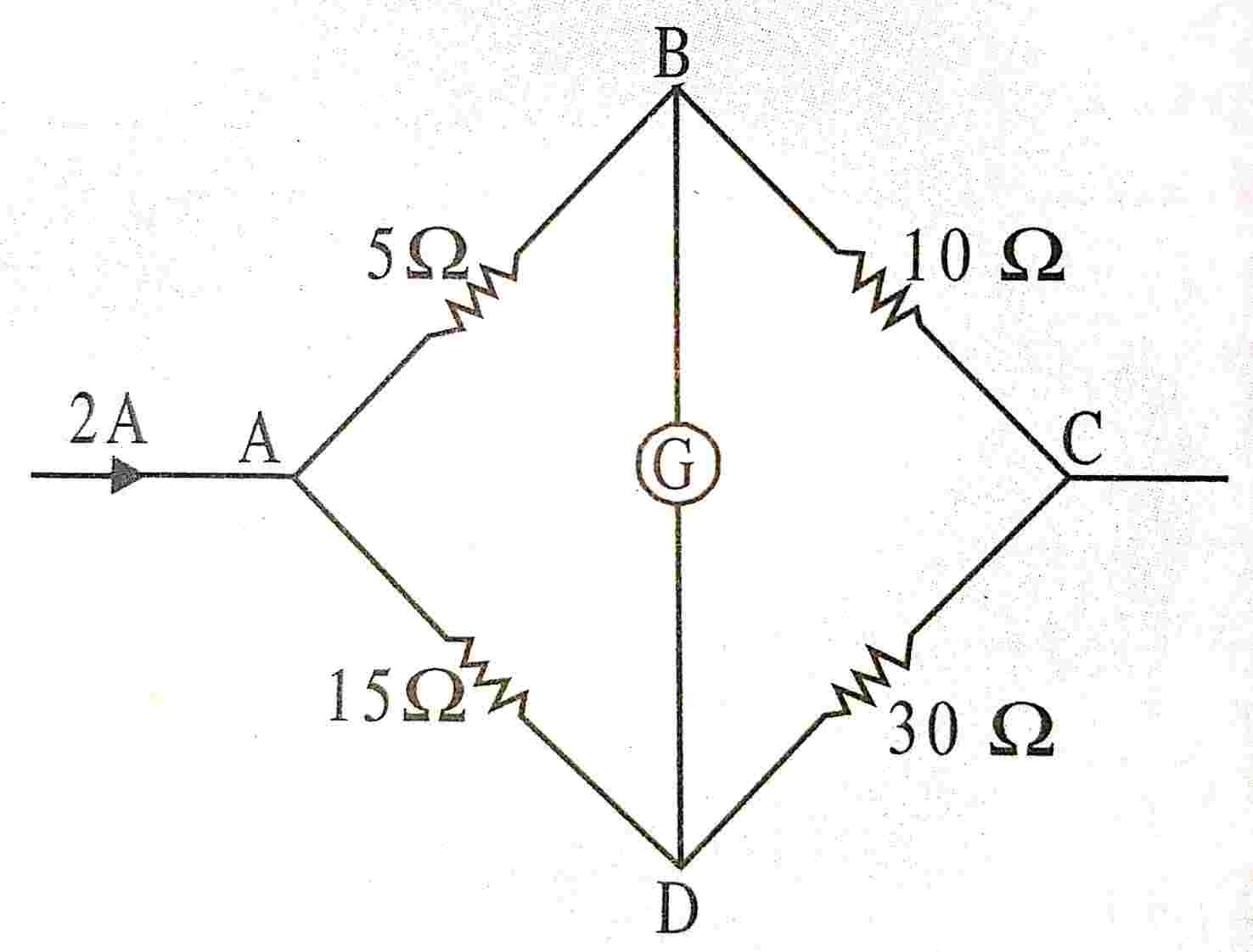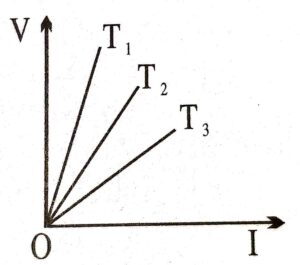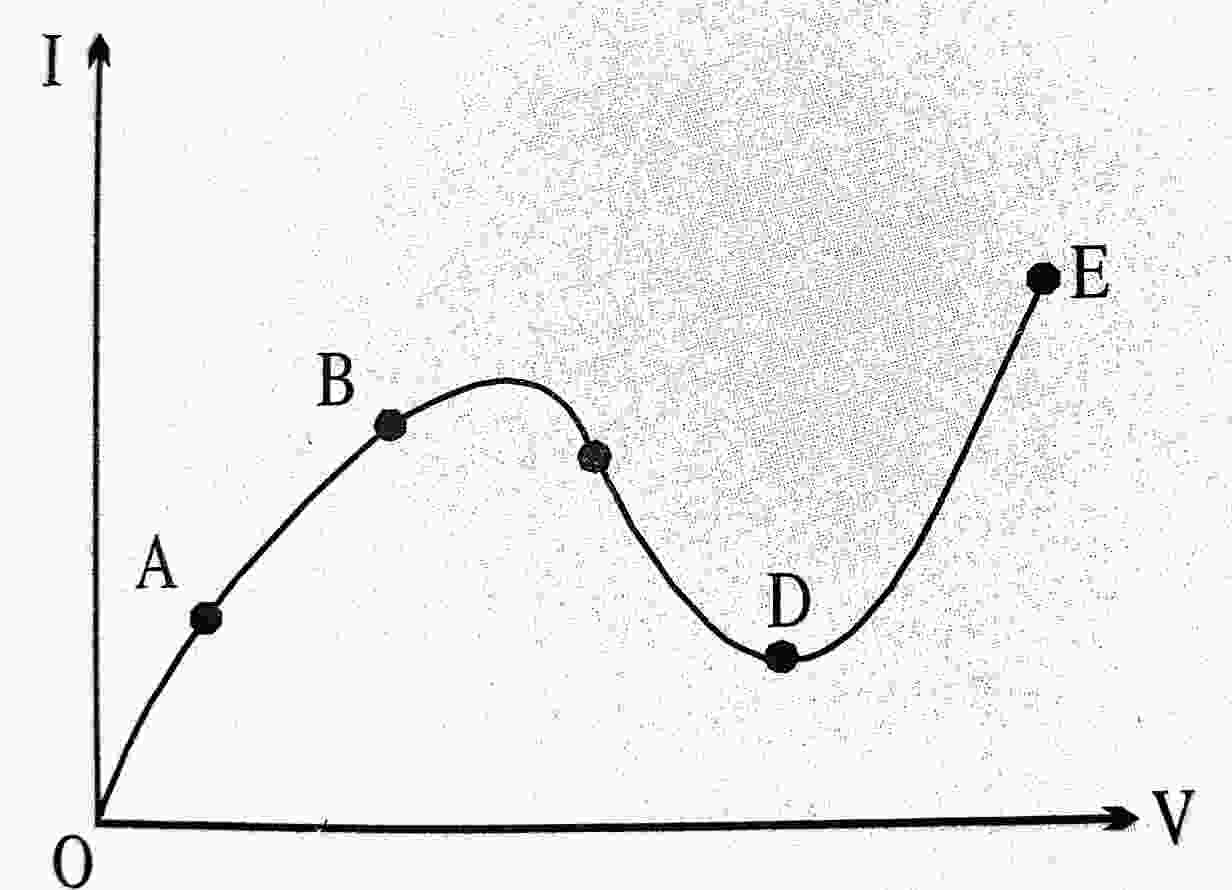CURRENT ELECTRICITY

1. The speed at which the current travels in a conductor is nearly :

(A) 3 x 104 ms-1
(B) 3 x 106 ms-1
(C) 3 x 108 ms-1
(D) 3 x 1010 ms-1

2. The resistance of a human body is about :

(A) 12 Ω
(B) 120 Ω
(C) 12 KΩ
(D) 120 MΩ

3. The number of electrons that constitute 1 A of current is :

(A) 6.25 x 1016
(B) 6.25 x 1017
(C) 6.25 x 1018
(D) 6.25 x 1019

4. How many different resistances are possible with two equal resistors ?

(A) 2
(B) 3
(C) 4
(D) 5

5. Given three equal resistors, how many different combinations (taken all of them together) can be made ?

(A) 3
(B) 4
(C) 5
(D) 6

6. Specific resistance of all metals is mostly affected by :

(A) temperature
(B) pressure
(C) magnetic field
(D) volume

7. The example of a non-ohmic resistance is :

(A) copper wire
(B) filament lamp
(C) carbon resistor
(D) diode

8. The length of a conductor is halved. Its resistance will be :

(A) halved
(B) doubled
(C) unchanged

9. In the above question, the conductance :

(A) halved
(B) doubled
(C) unchanged

10. Siemen is the unit of :

(A) resistance
(B) conductance
(C) specific conductance
(D) None of these

11. Two wires of copper are of the same length but have different diameters. When they are connected in series across a battery, the heat generated is H1 When connected in parallel across the same battery, the heat generated during the same time is H2 Then :

(A) H1 = H2
(B) H1 < H2
(C) H1 > H2
(D) H1 > H2

12. In questions 135, when the wires are connected in series, the heat produced in the thinner wire is H1 and that in the thicker wire is H2 Then :

(A) H1 = H2
(B) H1 < H2
(C) H1 > H2
(D) H1 ≥ H2

13. Kirchhoff’s first and second laws for electrical circuits are consequences of :

(A) conservation of energy
(B) conservation of electrical charge and energy respectively
(C) conservation of electric charge
(D) neither conservation of energy nor electric charge

14. A potential difference of 10 V is applied across a conductance of 2 S. The current in the conductor will be ;

(A) 20 A
(B) 5 A
(C) 0.2 A
(D) None of these

15. Ohm’s law is valid when the temperature of conductor is :

(A) very low
(B) very high
(C) varying
(D) constant

16. The emf of a car battery is about :

(A) 2.5 V
(B) 4.5 V
(C) 8.6 V
(D) 12 V

17. Emf is measured in :

(A) joule
(B) joule/coulomb
(C) joule-coulombs
(D) joule/coulomb/metre

18. 1 kilowatt hour is commonly known as:

(A) unit
(C) 1 curie
(D) None of these

19. How many joules are equal to 1 kWh ?

(A) 3.6.x 104
(B) 3.6 x 105
(C) 3.6 x 106
(D) None of these

20. Two bulbs 25 W, 220 V and 100 W, 220 V are given. Which has higher resistance ?

(A) 25 W
(B) 100 W
(C) both bulbs have equal resistance
(D) resistance of bulbs can not be compared

21. In the following figure represents a balanced Wheatstone bridge circuit. What is the value of X ?(A) 15 Ω
(B) 20 Ω
(C) 25 Ω
(D) 30 Ω

22. Potentiometer measures the potential difference more accurately than a voltmeter because :

(A) It has a wire of high resistance
(B) It has a wire of low resistance
(C) It does not draw current from external circuit
(D) It draws heavy current from external circuit

23. The sequence of colour bands of a carbon resistance of 47 kΩ will be :

(A) yellow, violet, red
(B) yellow, violet, orange
(C) violet, yellow, orange
(D) red, red, violet

24. The colour code for a resistance of 35 kΩ with 5% tolerance is :

(A) Orange, green, orange and gold
(B) Red, yellow, black and gold
(C) Orange, green, orange and silver
(D) Orange, green, red and gold

25. In the figure, shows a wheatstone bridge circuit. Its 2 Acurrent enters A, then what is the current in the arm BC ?(A) 3/2 A
(B) 1/2 A
(C) 2/3 A
(D) None of these

26. The resistivity of a conductor depends upon its :

(A) length
(B) area of cross-section
(C) resistance
(D) None of these

27. Internal resistance of a cell does not depend upon :

(A) electrode separation
(B) electrode material
(C) electrolyte
(D) electrolyte area

28. For which of the following dependence of drit velocity Vd on electric field E is ohm’s law obeyed ?

(A) vd = √E
(B) vd ∝ E
(C) vd = E2
(D) vd = constant

29. The voltage (V) and current (I) graph for a conductor at three different temperature T1, T2 and T3 are shown in the following figure. It follows:(A) T1 > T2 > T3
(B) T1 = T2 = T3
(C) T1 < T2 < T3
(D) T1 = T2 ≈ T3

30. If eight identical cells, each having an e.m.f. of 6V are connected in parallel, then the e.m.f. of the combination is :

(A) 1 V
(B) 1/8 V
(C) 48 V
(D) 6 V

31. Which part of the curve shown in the fig. represents the negative resistance ?(A) AB
(C) CD
(B) BC
(D) DE